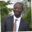Related Tags

javascript
math
array
communitycreator

# How to find the symmetric difference of two arrays in JavaScriptAbel Lifaefi Mbula

### Overview

Let's learn a bit about mathematics and programming today. It won't be too complicated.

### Symmetric difference

Let's start by learning the mathematical concept of symmetric difference.

Given two sets A and B, the symmetric difference between them, denoted as AB, is the set of elements that are in A or B, but not in A and B. In other words, it is the union of A and B, minus their intersection.

### Mathematical formula

The mathematical formula for this is: A△B = (A ∪ B) ∖ (A ∩ B)

### Example

The symmetric difference of {3, 4, 9} and {1, 2, 3} is {1, 2, 4, 9}.

### Venn diagram

This is how we can represent the symmetric difference:

Venn diagram: symmetric difference of A and B

### Code

Now that we have a better understanding of symmetric difference, let's see how we can implement it in JavaScript.

### Step 1: Initiate the function

We have the given array1 [1, 2, 3, 4] and array2 [1, 2, 3]. We return an array comprised of the symmetric difference of array1 and array2, which is .

function symmetricDiff(arr1, arr2) {
const newArr = []

return newArr
}

console.log(symmetricDiff([1, 2, 3, 4], [1, 2, 3]))
Initiate the function

As we can see in the code widget above, we have created a function called symmetricDiff() which accepts two array parameters, arr1 and arr2. In line 7, we call the function and print the output on the console.

### Step 2: Return the union

As per the symmetric difference definition, let's first find the union of arr1 and arr2.


function symmetricDiff(arr1, arr2) {
// union of two arrays
const union = [...arr1, ...arr2]

return union
}

console.log(symmetricDiff([1, 2, 3, 4], [1, 2, 3]))
Return the union

We used the spread syntax (aka spread operator) to concatenate the two arrays in one array called union. But, mathematically thinking, this won't give us the union we want because we'll have duplicated elements.

### Step 3: Return union without duplication

To fix this, we have to use a Set that only contains unique elements.


function symmetricDiff(arr1, arr2) {
const union = [...new Set(arr1, ...arr2)]

return union
}

console.log(symmetricDiff([1, 2, 3, 4], [1, 2, 3]))
Return union without duplication

Now that we have non-duplicate union elements as union, how about the intersection?

### Step 4: Return intersection

As we may already know, the intersection gives us the elements that arr1 and arr2 share in common. In our case, they are [1, 2, 3].


function symmetricDiff(arr1, arr2) {
const intersection = arr1.filter(i => arr2.includes(i))

return intersection
}

console.log(symmetricDiff([1, 2, 3, 4], [1, 2, 3]))
Return intersection

In line 3, we use filter() and includes() to find the intersection.

• filter() creates a new array with all elements that pass the test
• includes() checks if an array contains a given element in its entries

So, in our case, in line 3 filter() only creates an array with arr1 elements that exit in arr2.

### Step 5: Return the difference

Now that we have two new arrays, union and intersection, let's find the difference between them.


function symmetricDiff(arr1, arr2) {
// The union
const union = [...new Set(arr1, ...arr2)]

// The intersection
const intersection = arr1.filter(i => arr2.includes(i))

// The difference
const difference = union.filter(i => !intersection.includes(i))

return difference
}

console.log(symmetricDiff([1, 2, 3, 4], [1, 2, 3]))
Return the difference

That is it. We can now print out the symmetric difference following the definition we saw in the beginning.

Let's improve our code.


function symmetricDiff(arr1, arr2) {
const symmetricDiff = arr1
.filter(i => !arr2.includes(i))
.concat(arr2.filter(i => !arr1.includes(i)))

return symmetricDiff
}

console.log(symmetricDiff([1, 2, 3, 4], [1, 2, 3]))
Return the symmetric difference

Notice how we use concat() to concatenate the array made of elements of arr1 that are not in arr2 and the array made of elements of arr2 that are not in arr1.

RELATED TAGS

javascript
math
array
communitycreator

CONTRIBUTORAbel Lifaefi Mbula
RELATED COURSES

View all Courses

Keep Exploring

Learn in-demand tech skills in half the time